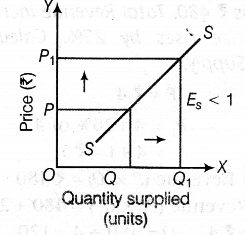# Using diagrams, explain various degrees of Price Elasticity of Supply

The various degrees of Price Elasticity of Supply are given below:
(i) Perfectly elastic supply In this case, a slight change in price causes infinite change in quantity supplied. The supply curve SS is parallel to X-axis and {{E}_{s}} = 0 as shown in the figure: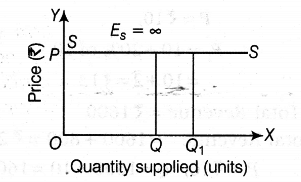(ii) Perfectly inelastic supply It is a situation where the quantity supplied remains unchanged, whatever be the changes in price. Hence, the supply curve is parallel to 7-axis and {{E}_{s}} = 0, as shown in the figure: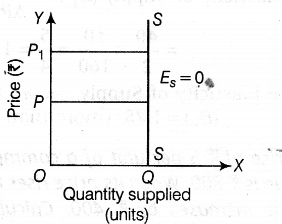(iii) Unitary elastic supply In this case, percentage change in quantity supplied is exactly equal to percentage change in price. Hence, the supply curve is a straight line originating from the origin and sloping upward and {{E}_{s}} = 1, as shown in the figure: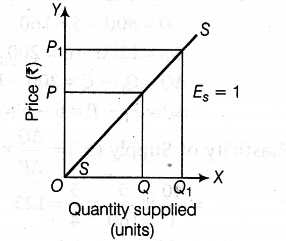(iv)More than unitary elastic supply In this situation, percentage change in quantity supplied is greater than the percentage change in price.An upward sloping straight line supply curve originates from 7-axis and {{E}_{s}}> 1, as shown in the figure: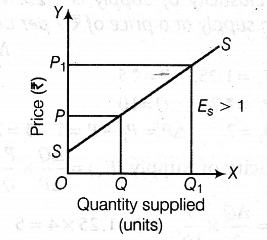(v) Less than unitary elastic supply In this situation, percentage change in quantity supplied is less than percentage change in price. An upward sloping straight line supply curve originates from X-axis and {{E}_{s}}<1, as shown in the figure: